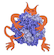IMP  2.4.0 The Integrative Modeling Platform
algebra/utility.h File Reference

Functions to deal with very common math operations. More...

#include <cmath>
#include <IMP/algebra/algebra_config.h>
#include <IMP/base/exception.h>Include dependency graph for algebra/utility.h:This graph shows which files directly or indirectly include this file:

Go to the source code of this file.

## Namespaces

IMP
Synonym for IMP::kernel.

IMP::algebra
General purpose algebraic and geometric methods that are expected to be used by a wide variety of IMP modules.

## Functions

bool IMP::algebra::get_are_almost_equal (const double a, const double b, const double epsilon)
Compares two values (intended for doubles) More...

double IMP::algebra::get_closer_power_of_2 (double x)
Closest power of 2 for a number, not necessarily higher. More...

template<typename T >
IMP::algebra::get_constrained (const T x, const T x0, const T xF)
Constrains a value between two given limits. More...

float IMP::algebra::get_next_larger_power_of_2 (float x)
Closest power of 2 that can contain a number x. More...

double IMP::algebra::get_next_larger_power_of_2 (double x)
Closest power of 2 that can contain a number x. More...

template<typename T >
int IMP::algebra::get_rounded (const T &x)
Rounds a number to next integer. More...

template<typename T >
int IMP::algebra::get_sign (const T &x)
Sign of a number. 1 if the number is higher or equal to 0 and -1 otherwise. More...

double IMP::algebra::get_squared (double x)

## Detailed Description

Functions to deal with very common math operations.